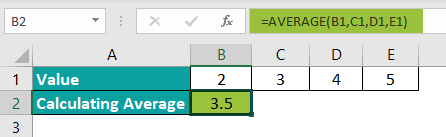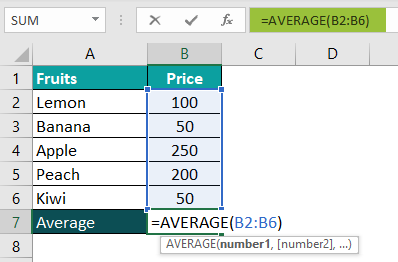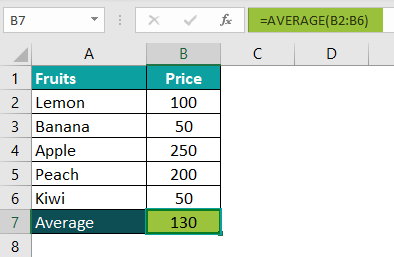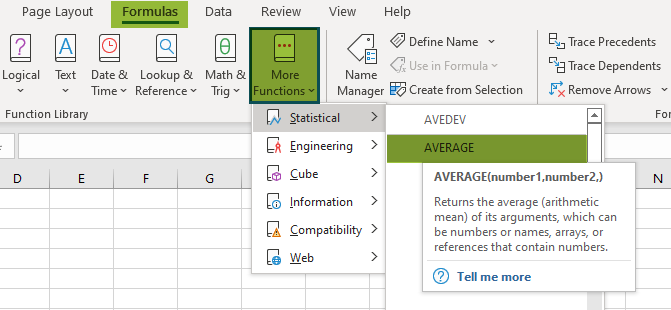# AVERAGE Excel FunctionArticle byExcelMojo Team## What is AVERAGE Function in Excel?

The AVERAGE function in Excel calculates the arithmetic mean of the numbers passed in the arguments. The arguments can be direct numbers or cell references containing numbers. It is an inbuilt Statistical Excel function.

For example, the following image depicts three numbers – 10, 20, and 30 in column A. We will get the average of the numbers using the AVERAGE formula in cell A5:

=AVERAGE(A2:A4)

The result comes as 20.

###### Key Takeaways
• The AVERAGE Excel formula is a Statistical Excel function that users can use to get the average (arithmetic mean) of numeric values, cell references, ranges, or all three combined in Excel.
• The syntax of the AVERAGE function is =AVERAGE(number1,[number2],…), where “number1” is a mandatory argument and “number2” is an optional argument. They are the values to get the average.
• The AVERAGE Excel function automatically updates when we include more rows and columns or delete any rows and columns.
• The function makes output as easy to understand in numerical form, eliminates the difficulties of manually adding and calculating the average of the values, ignores the empty cells and text values, and adds all cell ranges, whether they are continuous or not.

### AVERAGE() Excel Syntax

Below is the formula of Average() in excel –

• number1 = The first numeric value for which the average is calculated. It is the mandatory argument.
• number2 = The subsequent numeric for which the average is to be calculated. It is the optional argument.

### How To Use AVERAGE Function?

We can use AVERAGE function in Excel in two ways:

1. Choose the empty cell which will contain the result.
2. Click the “Formulas.”
3. Select “More Functions” from the drop-down menu.
4. Click on “Statistical” from the drop-down menu.
5. Select “AVERAGE” from the drop-down menu.
6. A window called “Function Arguments” appears.
7. As the number of arguments, enter values in the “Number 1” and “Number 2” arguments.
8. Select OK.

#### 2. Manually On The Sheet

• Select the empty cell
• Type =Average(
• Double click on the AVERAGE command
• Select the range of the cell or array
• Press “Enter.”

The image below depicts a series of numbers – 2, 3, 4, and 5. So, let us get the average of the numbers with the AVERAGE Excel function.

In the table, the data is reflected as below:

• Row 1 shows Values – 2, 3, 4, 5
• Cell B2 calculates the Average

The steps to calculate AVERAGE in excel of the given numbers are as follows:

1. First, we will choose the cell where we want the result to show up. Cell B2 would be the cell in this case.

2. Next, we will enter the AVERAGE formula in cell B2.

3. Select the cell references from the table, i.e., B1,C1,D1,E1.

=AVERAGE(B1,C1,D1,E1)4. After entering each value in the preceding step, press the “Enter” key. You will get the result in cell B2 as 3.5 in the image below.Therefore, the manual calculation of the average of the given values is as follows:

SUM = 2+3+4+5 = 14

AVERAGE = {SUM/Number of values}

= 14/4

= 3.5

### Examples

Let us look at some advanced examples to understand how the AVERAGE Excel function works:

#### Example #1 – AVERAGEA Function in Excel

The AVERAGEA function is the predefined and family member of the AVERAGE Excel function. It counts all the cells in the range with numbers, strings, and Boolean values.

The formula of the AVERAGEA function is as follows:

=AVERAGEA(value1,[value2],…)

The image below shows multiple values types: fruits names, numbers, and Boolean values. Here, we will get the average of these values using the AVEARGEA function.

In the table, the data is reflected as below:

• Column A shows Values

The steps to calculate AVERAGE in excel of the given values are as follows:

Step 1:  First, we will choose the cell where we want the result to show up. Cell B2 would be the cell in this case.

Step 2:  Next, we will enter the AVERAGEA formula in cell B2.

Step 3: Select the value1, which is the range of cells from the starting cell address to the ending cell address of the table, i.e., A2:A8. The complete formula will be:

=AVERAGEA(A2:A8)

Step 4: After entering each value in the preceding step, press the “Enter” key. You will get the result in cell B2 as 8.714 in the image below.

Therefore, the AVERAGEA function also calculates the average of text strings and Boolean values. The text string’s actual value is ‘0’, the value of ‘TRUE’ is ‘1’, and the value of ‘FALSE’ is ‘0’.

The manual calculation of the average of the given values is as follows:

SUM = 0+0+10+20+30+1+0 = 61

AVERAGEA = {SUM/Number of values}

= 61/7

= 8.714

#### Example #2 – AVERAGEIF Function in Excel

The AVERAGEIF function, like the AVERAGEA function, is also a predefined and family member of the AVERAGE Excel function. It counts all the cells in the range as specified by the conditions.

The formula of the AVERAGEIF function is as follows:

=AVERAGEIF(range,criteria,[average_range])

The image below shows items and their sales. We will get the average per the criteria given for these values using the AVEARGEIF function.

In the table, the data is reflected as below:

• Column A shows Items
• Column B contains Sales

The steps to calculate AVERAGE in excel of the given values are as follows:

Step 1: First, we will choose the cell where we want the result to show up. Cell C2 would be the cell in this case.

Step 2: Next, we will enter the AVERAGEIF formula in cell C2.

Step 3: Select the value, which ranges from the starting cell address to the ending cell address of the table, i.e., A2:A9.

Step 4: Set the criteria we want to count, i.e., “Lemon Tart.”

Step 5: Select the average range, which is the range from the starting cell address to the ending cell address of the table, i.e., B2:B9.

Step 6: After entering each value in the preceding step, press the “Enter” key. You will get the result is shown in cell C2 as 130 in the image below.

Therefore, the AVERAGEIF function calculates the average as per the criteria given. In the manual calculation of the average, when the criteria are checked, the value of “Lemon Tart” is as follows:

SUM = 100+200+90 = 390

AVERAGE = {SUM/Number of values}

= 390/3

= 130

#### Example #3 – AVERAGEIFS Function in Excel

The AVERAGEIFS function, like the AVERAGEA and AVERAGEIF functions, is also a predefined and family member of the AVERAGE Excel function. It calculates the average of the cells in the given average range as specified by the range and the criteria.

The formula of the AVERAGEIFS function is as follows:

=AVERAGEIFS(average_range,criteria_range1,criteria1,….)

The image below shows the region, month, and sales. We will get the average per the criteria given for these values using the AVEARGEIFS function.

In the table, the data is reflected as below:

• Column A shows Region
• Column B contains the Month
• Column C contains Sales

The steps to get the average of the given values are as follows:

Step 1:  First, we will choose the cell where we want the result to show up. Cell C8 would be the cell in this case.

Step 2:  Next, we will enter the AVERAGEIFS formula in cell C8.

Step 3: Select the value, which is the average range from the starting cell address to the ending cell address of the table, i.e., C2:C7.

Step 4: Select the value, which is the criteria range1 from the starting cell address to the ending cell address of the table, i.e., A2:A7.

Step 5: Set the criteria1 we want to count, i.e., North.

Step 6: After entering each value in the preceding step, press the “Enter” key. You will get the result is shown in cell C8 as 1656.5 in the image below.

Therefore, the AVERAGEIFS function calculates the average as per the average range, criteria range, and criteria. In the manual calculation of the average, when the criteria are checked, the value of “North” is as follows;

SUM = 1580+1733 = 3313

AVERAGE = {SUM/Number of values}

= 3313/2

= 1656.5

### Important Things To Note

• The AVERAGE Excel function automatically ignores the cells that contain a text string.
• The “#DIV/0!” error in the AVERAGE Excel function occurs when the range consists of the text string.
• The “#VALUE!” error in the AVERAGE Excel function occurs when the formula’s text value is entered directly.
• The AVERAGE Excel function automatically ignores empty cells.
• The function ignores logical values, i.e., Boolean values and cells containing error values.
• The AVERAGE Excel function does not work on text values.
• The function can handle up to 255 characters.

What is the AVERAGE function in Excel?

The AVERAGE function in Excel returns the average of the numerical values. The values can be numbers, percentages, or times. The purpose of using this function is to calculate the mean of the list. It first calculates the sum of the list’s values and divides it by the number of values on the list.

=AVERAGE(number1,[number2],…)

How to use Excel AVERAGE function?

Below is the step-by-step process of calculating the average of the numbers using the AVERAGE Excel function:
1. Select the empty cell
2. Type =Average(
3. Double click on the AVERAGE command
4. Select the range of the cell or array
5. Press “Enter.”

For example, the following image depicts the fruits in column A and their prices in column B. We will get the average of the numbers using the AVERAGE formula in the cell B7:
=AVERAGE(B2:B6)The result comes as ‘130’.Where is AVERAGE function in Excel?

One can activate the AVERAGE function in Excel using the following steps:
1. Choose the empty cell which will contain the result.
2. Go to the “Formulas” tab and click it.
3. Select “More Functions” from the drop-down menu.
4. Select “Statistical” from the drop-down menu.
5. Select “AVERAGE” from the drop-down menu.
6. A window called “Function Arguments” appears.
7. As the number of arguments, enter the values in the “Number 1” and “Number 2” arguments.
8. Select OK.